# 一种变阻尼稳定伞的气动分析Aerodynamic Analysis of a Variable Damping Stabilized Parachute

• 全文下载: PDF(2661KB)    PP.31-37   DOI: 10.12677/MOS.2019.81005
• 下载量: 401  浏览量: 1,054

The hose-paradrogue is prone to swing motion due to the interference of airflow during air refueling, which makes the docking process more difficult. The flexible hose-paradrogue system in flexible aerial refueling is simulated and analyzed by Computational Fluid Dynamics simulation technology, which provides reference for the design of flexible hose-cone sleeve controller and improves the controllability of flexible hose-cone sleeve system.

1. 引言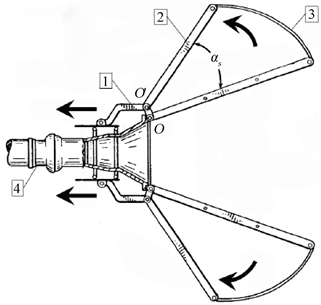Figure 1. Structural sketch of a variable damping stabilization umbrella

2. 变阻尼稳定伞设计及伞面形状的确定

3. CFD数值模拟

3.1. 稳定伞模型

3.2. 仿真结果分析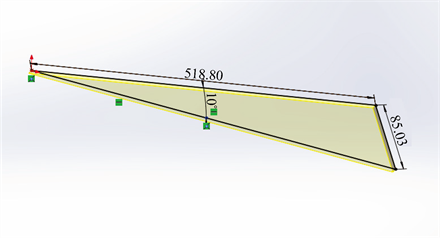(a)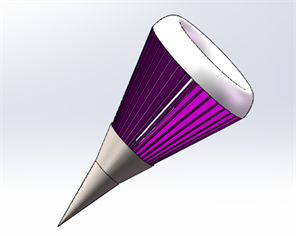(b)

Figure 2. (a) 10-degree umbrella support size; (b) SolidWorks model of paradrogue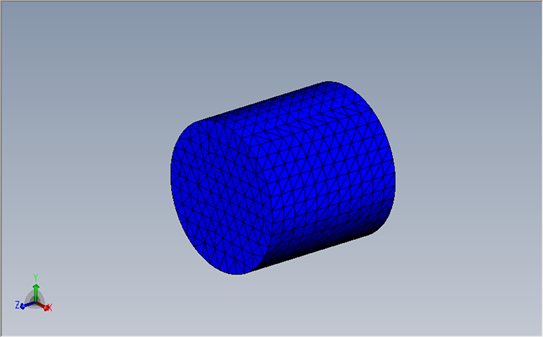Figure 3. The computational domain of steady parachute flow field(a) αs = 10˚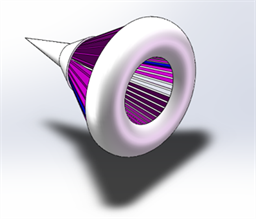(b) αs = 20˚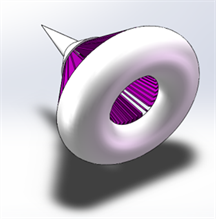(c) αs = 30˚

Figure 4. The shape of three conical sleeve stabilized parachutes

$\text{T}={\text{T}}_{\text{a}}-0.0065H$ (1)

$\frac{\text{p}}{{\text{P}}_{\text{a}}}={\left(\frac{\text{T}}{{\text{T}}_{a}}\right)}^{5.25588}$ (2)

$\frac{\rho }{{\rho }_{\text{a}}}={\left(\frac{\text{T}}{{\text{T}}_{a}}\right)}^{4.25588}$ (3)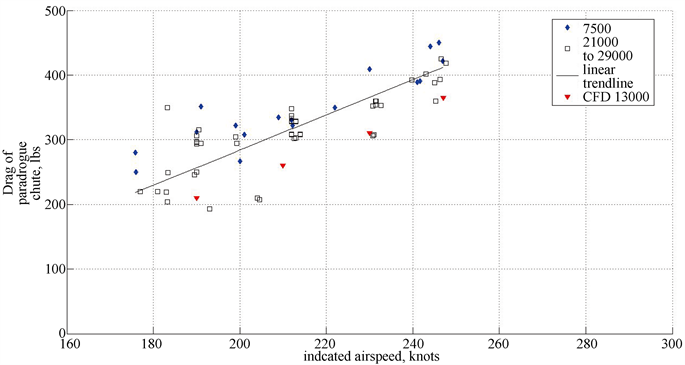Figure 5. Comparison of CFD simulation results with flight test data

4. 模拟结果与分析

$\text{D=}\frac{1}{2}{\rho }_{\infty }{\text{V}}_{\infty }{}^{2}{\text{S}}_{\text{ref}}\text{Cd}$ (4)

$\text{Cd=}\mathrm{f}\left({\text{V}}_{\infty },{\alpha }_{s}\right)$ (5)

$\text{Cd}\approx \text{f}\left({\alpha }_{\text{s}}\right)$ (6)

$\text{Cd}\approx \text{A}{\alpha }_{\text{s}}{}^{2}+B{\alpha }_{\text{s}}+C$ (7)

$\text{Cd}\approx 0.0003{\alpha }_{\text{s}}{}^{2}-\text{0}\text{.003}{\alpha }_{\text{s}}+0.65$ (8)(a) ${\alpha }_{s}$ = 10˚(b) ${\alpha }_{s}$ =20˚(c) ${\alpha }_{s}$ = 10˚

Figure 6. Ma = 0.5, streamline diagram of leeward section of stable umbrella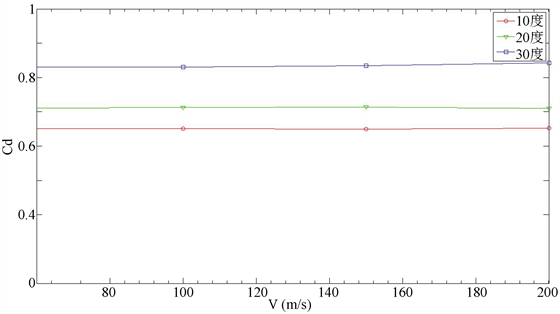Figure 7. Resistance coefficient-velocity curve of stable parachute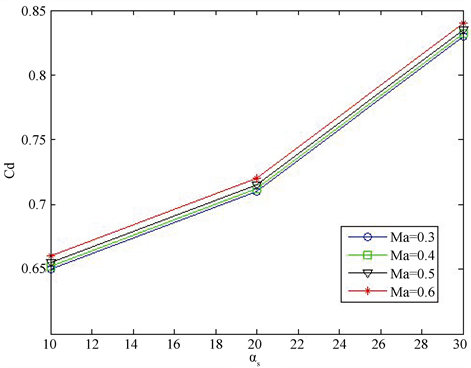Figure 8. The relationship curve between drag coefficient and brace angle of stabilized parachute

5. 总结

  Ro, K. and Basaran, E. (2006) Aerodynamic Investigations of Paradrogue Assembly in Aerial Refueling System. AIAA Aerospace Sciences Meeting & Exhibit, 68-70.  王海涛, 董新民, 窦和锋, 等. 软管锥套式空中加油系统建模与特性分析[J]. 北京航空航天大学学报, 2014, 40(1): 92-98.  张进, 袁锁中, 龚全铨. 空中加油软管-锥套动态建模及其飘摆运动抑制[J]. 科学技术与工程, 2015, 15(8): 134-139.  Williamson, W.R., Reed, E., Glenn, G.J., et al. (2010) Controllable Drogue for Automated Aerial Refueling. Journal of Aircraft, 47, 515-527. https://doi.org/10.2514/1.44758  Kirkland, W.L. (2010) Variable Speed Drogue US2011/0108669.  Ro, K., Ahmad, H. and Kamman, J. (2009) Dynamic Modeling and Simulation of Hose-Paradrogue Assembly for Mid-Air Operations. AIAA Journal, Seattle, Washington, 6-9 April 2009, 1-14.  吴成林. 空中加油软管—锥套系统动态特性研究[D]: [硕士学位论文]. 南京: 南京航空航天大学, 2015.  吴玲, 孙永荣, 黄斌, 等. 不确定环境下的软管-锥套建模及控制研究[J]. 计算机工程与应用, 2017(18): 250-256.  Ro, K. and Kamman, J.W. (2010) Modeling and Simulation of Hose-Paradrogue Aerial Refueling Systems. Journal of Guidance, Control, and Dynamics, 33, 53-63. https://doi.org/10.2514/1.45482  张进, 袁锁中, 龚全铨, 等. 空中加油软管-锥套空中飘摆运动建模与控制[J]. 系统仿真学报, 2016, 28(2): 388-395.